# Unscented Kalman Filters¶

In :
#format the book
%matplotlib inline
from __future__ import division, print_function
import matplotlib.pyplot as plt
import book_format

Out:

In the last chapter we discussed the difficulties that nonlinear systems pose. This nonlinearity can appear in two places. It can be in our measurements, such as a radar that is measuring the slant range to an object. Slant range requires you to take a square root to compute the x,y coordinates:

$$x=\sqrt{slant^2 - altitude^2}$$

The nonlinearity can also occur in the process model - we may be tracking a ball travelling through the air, where the effects of gravity and air drag lead to highly nonlinear behavior. The standard Kalman filter performs poorly or not at all with these sorts of problems.

In the last chapter I showed you a plot like this. I have altered the equation somewhat to emphasize the effects of nonlinearity.

In :
from nonlinear_plots import plot_transfer_func
from numpy.random import normal
import numpy as np

data = normal(loc=0.0, scale=1, size=500000)

def g(x):
return (np.cos(4*(x/2+0.7)))-1.3*x

plot_transfer_func (data, g, lims=(-3.5,3.5), num_bins=300)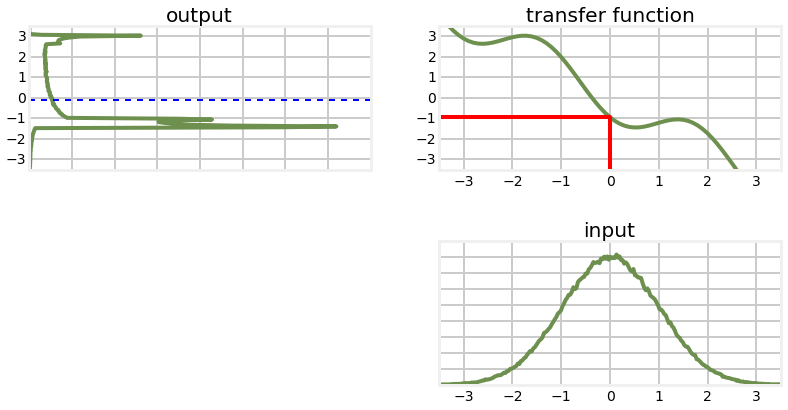I generated this by taking 500,000 samples from the input, passing it through the nonlinear transform, and building a histogram of the result. From the output histogram we can compute a mean and standard deviation which would give us an updated, albeit approximated Gaussian.

It has perhaps occurred to you that this sampling process constitutes a solution to our problem. Suppose for every update we generated 500,000 points, passed them through the function, and then computed the mean and variance of the result. This is called a 'monte carlo' approach, and it used by some Kalman filter designs, such as the Ensemble filter. Sampling requires no specialized knowledge, and does not require a closed form solution. No matter how nonlinear or poorly behaved the function is, as long as we sample with enough points we will build an accurate output distribution.

"Enough points" is the rub. The graph above was created with 500,000 points, and the output is still not smooth. You wouldn't need to use that many points to get a reasonable estimate of the mean and variance, but it will require many points. What's worse, this is only for 1 dimension. In general, the number of points required increases by the power of the number of dimensions. If you only needed $50$ points for 1 dimension, you'd need $50^2=2,500$ for two dimensions, $50^3=125,000$ for three dimensions, and so on. So while this approach does work, it is very computationally expensive. The Unscented Kalman filter uses a similar technique but reduces the amount of computation needed by a drastic amount by using a deterministic method of choosing the points.

## Choosing Sigma Points¶

Let's look at the problem in terms of a 2D covariance ellipse. I choose 2D merely because it is easy to plot. Naturally this will extend to any number of dimensions. Assuming some arbitrary nonlinear function, we will take random points from the first covariance ellipse, pass them through the nonlinear function, and plot their new position.

In :
import ukf_internal
ukf_internal.show_2d_transform()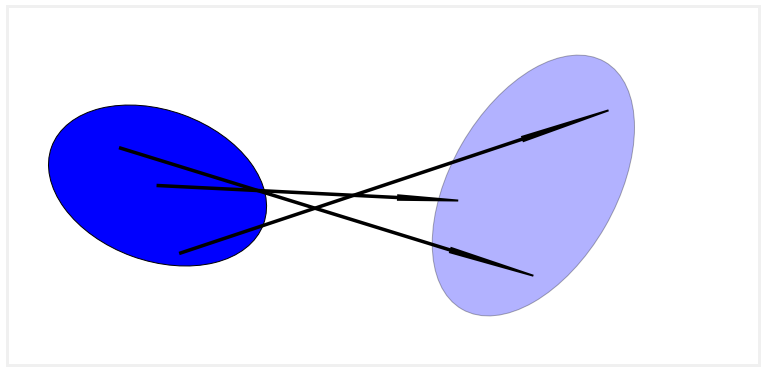Here on the left we show an ellipse depicting the $1\sigma$ distribution of two state variables. The arrows show how three randomly sampled points might be transformed by some arbitrary nonlinear function to a new distribution. The ellipse on the right is drawn semi-transparently to indicate that it is an estimate of the mean and variance of this collection of points - if we were to sample, say, a million points the shape of the points might be very far from an ellipse.

Let's look at that by running a bunch of points through a nonlinear function. We will write a function to pass randomly generated points with a Gaussian distribution through the system

\begin{aligned}x&=x+y\\ y &= 0.1x^2 + y^2\end{aligned}

for the mean and covariance $$\mu = \begin{bmatrix}0\\0\end{bmatrix}, \Sigma=\begin{bmatrix}32&15\\15&40\end{bmatrix}$$

In :
from numpy.random import randn
import numpy as np
from numpy import sin, cos, tan,log
import matplotlib.pyplot as plt

from numpy.random import multivariate_normal

def f(x,y):
return x+y, .1*x**2 +y*y

mean = (0, 0)
p = np.array([[32, 15],[15., 40.]])

xs ,ys = multivariate_normal(mean=mean, cov=p, size=50000).T
fxs, fys = [], []
for x,y in zip(xs,ys):
fx, fy = f(x,y)

fxs.append(fx)
fys.append(fy)

# Compute mean
mean_fx = f(*mean)

computed_mean_x = np.average(fxs)
computed_mean_y = np.average(fys)

plt.subplot(121)
plt.scatter (xs,ys, marker='.', alpha=0.1)
plt.axis('equal')

plt.subplot(122)
plt.scatter(fxs, fys, marker='.', alpha=0.1)
plt.scatter(mean_fx, mean_fx,
marker='v', s=300,c='k', label='Linearized Mean')
plt.scatter(computed_mean_x, computed_mean_y,
marker='*',s=120,c='r', label='Computed Mean')
plt.ylim([-10,290])
plt.xlim([-150,150])
plt.legend(loc='best', scatterpoints=1)
plt.show()
print ('Difference in mean x={:.3f}, y={:.3f}'.format(
computed_mean_x-mean_fx, computed_mean_y-mean_fx))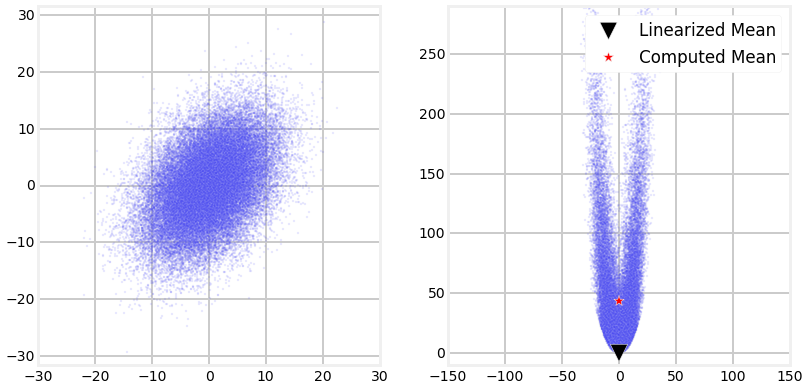Difference in mean x=0.020, y=43.466


This plot shows the strong nonlinearity that occurs with this function, and the large error that would result if we linearized the function at (0,0).

We used 5,000 points to generate this solution. While the computed mean is quite accurate, computing 5,000 points for every update would cause our filter to be very slow. So, what would be fewest number of sampled points that we can use, and what kinds of constraints does this problem formulation put on the points? We will assume that we have no special knowledge about the nonlinear function as we want to find a generalized algorithm that works for any function. For reasons that come clear in the next section, we will call these points sigma points.

Let's consider the simplest possible case and see if it offers any insight. The simplest possible system is identity - the transformation does not alter the input. In mathematical notation this is just $f(x) = x$. It should be clear that if our algorithm does not work for the identity function then the filter will never converge. In other words, if the input is 1 (for a one dimensional system), the output must also be 1. If the output was different, such as 1.1, then when we fed 1.1 into the transform at the next time step, we'd get out yet another number, maybe 1.23. The filter would run away (diverge).

The fewest number of points that we can use is one per dimension. This is the number that the linear Kalman filter uses. The input to a Kalman filter for the distribution $\mathcal{N}(\mu,\sigma^2)$ is just $\mu$ itself. So while this works for the linear case, it is not a good answer for the nonlinear case.

Perhaps we can use one point per dimension, but altered somehow. However, if we were to pass some value $\mu+\Delta$ into the identity function $f(x)=x$ it would not converge, so this is not a possible algorithm. We must conclude that a one point sample will not work.

So, what is the next lowest number we can choose? Consider the fact that Gaussians are symmetric, and that we probably want to always have one of our sample points be the mean of the input for the identity function to work. Two points would require us to select the mean, and then one other point. That one other point would introduce an asymmetry in our input that we probably don't want. It would be very difficult to make this work for the identity function $f(x)=x$.

The next lowest number is 3 points. 3 points allows us to select the mean, and then one point on each side of the mean, as depicted on the chart below.

In :
ukf_internal.show_3_sigma_points()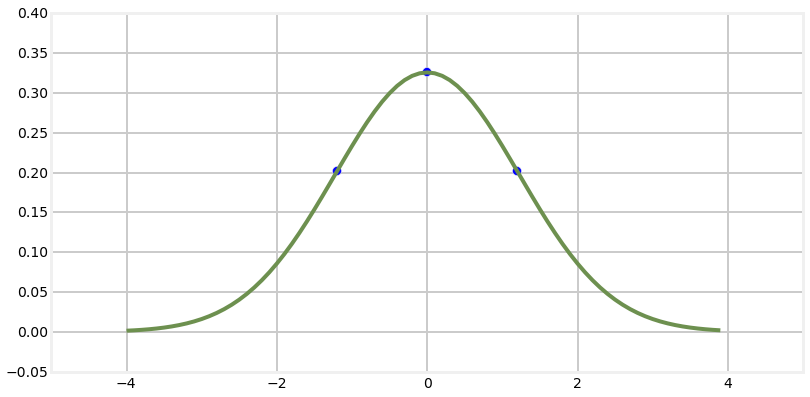For this to work for identity we will want the sums of the weights to equal one. We can always come up with counterexamples, but in general if the sum is greater or less than one the sampling will not yield the correct output. Given that, we then have to select sigma points $\mathcal{X}$ and their corresponding weights so that they compute to the mean and variance of the input Gaussian. So we can write

\begin{aligned} 1 &= \sum_i{w_i}\;\;\;&(1) \\ \mu &= \sum_i w_i\mathcal{X}_i\;\;\;&(2) \\ \Sigma &= \sum_i w_i{(\mathcal{X}_i-\mu)(\mathcal{X}_i-\mu)^\mathsf{T}}\;\;\;&(3) \end{aligned}

If we look at this is should be clear that there is no one unique answer - the problem is unconstrained. For example, if you choose a smaller weight for the point at the mean for the input, you could compensate by choosing larger weights for the rest of the $\mathcal{X}$, and vice versa if you chose a larger weight for it. Indeed, these equations do not require that any of the points be the mean of the input at all, though it seems 'nice' to do so, so to speak.

But before we go on I want to make sure the idea is clear. We are choosing 3 points for each dimension in our covariances. That choice is entirely deterministic. Below are three different examples for the same covariance ellipse.

In :
ukf_internal.show_sigma_selections()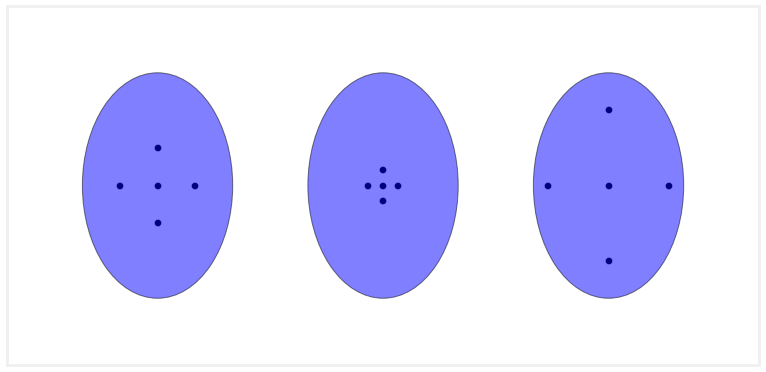Note that while I chose the points to lie along the major and minor axis of the ellipse, nothing in the constraints above require me to do that; however, it is fairly typical to do this. Furthermore, in each case I show the points evenly spaced; again, the constraints above do not require that. However, the technique that we develop in the next section does do this. It is a reasonable choice, after all; if we want to accurately sample our input it makes sense to sample in a symmetric manner.

There are several published ways for selecting the sigma points. For now I will stick with the original implementation by Julier and Uhlmann . This method defines a constant kappa ($\kappa$) which controls how spread out the sigma points are. Before we work through the derivation, let's just look at some examples. I will use the class UnscentedKalmanFilter from FilterPy to generate the sigma points for us. It is convention to write

import UnscentedKalmanFilter as UKF



because UKF is the standarized shorthand used in the literature for this filter.

I will plot the sigma points on top of a covariance ellipse showing the first and second deviations, and size them based on the weights assigned to them. I am using the covariance ellipse

$$\mathbf{P} = \begin{bmatrix} 4&2\\2&4\end{bmatrix}$$
In :
from numpy import array
import matplotlib.pyplot as plt
from filterpy.kalman import UnscentedKalmanFilter as UKF
from filterpy.common import plot_covariance_ellipse

x = array([0, 0])
P = array([[4, 2],
[2, 4]])

sigmas_kp5 = UKF.sigma_points(x=x, P=P, kappa=0)
sigmas_k2 = UKF.sigma_points(x=x, P=P, kappa=2)
W0 = UKF.weights(2, kappa=0)
W1 = UKF.weights(2, kappa=2)

for i in range(len(W0)):
p1 = plt.scatter(sigmas_kp5[i,0], sigmas_kp5[i,1], s=W1[i]*500, c='g')
p2 = plt.scatter(sigmas_k2[i,0], sigmas_k2[i,1], s=W0[i]*500, c='b')

plt.legend([p1, p2], ['Kappa = 0.5', 'Kappa = 2'])
plot_covariance_ellipse(x, P, facecolor='g', alpha=0.2, std=[1,2])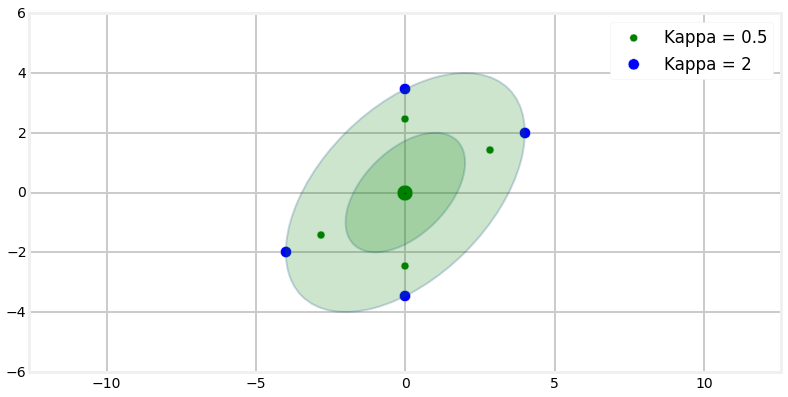We can see that the sigma points lie between the first and second deviation, and that the larger $\kappa$ spreads the points out further. Furthermore, the larger $\kappa$ weighs the mean (center point) higher than the smaller $\kappa$, and weighs the rest of the sigma points less. This should fit our intuition - the further a point is from the mean the less we should weight it. We don't know how these weights and sigma points are selected yet, but the choices look reasonable.

## Handling the Nonlinearities¶

I explained at the beginning of the chapter that there are two places for nonlinearities to occur. The first is in the state transition function, and the second is in the measurement function. For the linear Kalman filter we use the equation

$$\mathbf{x}^-=\mathbf{Fx}$$

to propogate the state from one epoch to the next. As you remember F defines a set of linear equations that performs this calculation. However, suppose we are tracking a ball or missile in flight. Air drag causes the behavior to be nonlinear, and therefore there are no linear equations that will compute the state.

The linear Kalman filter uses the measurement function in this equation

$$\hat{\mathbf{x}} = \mathbf{K}(\mathbf{z-Hx}^-)$$

to incorporate the measurements into the state. The term $\mathbf{Hx}$ converts $\mathbf{x}$ to a measurement using the linear equations implied by \mathbf{H}$. The UKF assumes that both the measurement function and state transition function are nonlinear, though in some problems only one or the other are nonlinear. If they are nonlinear we can not represent them with a matrix. Instead, we will define a function$h(x)$for the measurement function and$f(x)$for the state transition function. We will implement these as Python functions that take a state$x$and return either a state$x$for the state transition function, or a measurement vector$z$for the measurement function. The functions will look something like: def hx(x): z = # do something to compute z return z def fx(x, dt): x_est = x propagated forward in time by dt return x_est ## Tracking an Aircraft¶ So far we haven't been very concrete, so let's jump into solving a problem. In the Multivariate Kalman Filter chapter I presented an example of tracking an aircraft with two radar stations. This is a nonlinear problem so we were not able to construct a Kalman filter for that situation in that chapter. We are now able to do so. Assume we want to track an aircraft in 2 dimensions. We will ignore altitude to simplify the exposition and code. There are two radar stations on the ground, each which provides a range (straight line distance) and bearing to the aircraft from the station's position. The radars have a$1\sigma$error of$0.5^\circ$in the bearing measurement, and a$1\sigma$error of 0.1km for the range. We will assume that the aircraft does not engage in high dynamic flight, so we will chose a constant velocity process model for it. In other words, our state will contain the position and velocity of the aircraft in the$x$and$y$coordinates, like so. $$\mathbf{x} = \begin{bmatrix}x\\ \dot{x} \\ y \\ \dot{y}\end{bmatrix}$$ Now we need to design our state transition function. With a linear Kalman filter, for a constant velocity model we would set $$\bf{F} = \begin{bmatrix} 1&dt&0&0 \\ 0&1&0&0 \\ 0&0&1&dt \\ 0&0&0&1 \end{bmatrix}$$ so that$\bf{Fx}$computes the next position with the Newtonian equation$\bf{x}^- = \bf{x} + \dot{\bf{x}}\,\Delta t$. We are assuming linear behavior for the aircraft over short time periods in this problem so this formulation suffices. However FilterPy's UKF implementation is designed to handle nonlinear problems, so we need to implement this as a function. The state transition function has the signature def fx(x, dt)  where x is the state variable (a one dimensional numpy.array), and dt is a float specifying the time step. Note that the name fx is not required, you may name it anything you like. We might implement this function as In : def fx(x, dt): """ state transition function for a constant velocity aircraft""" F = np.array([[1, dt, 0, 0], [0, 1, 0, 0], [0, 0, 1, dt], [0, 0, 0, 1]]) return np.dot(F, x)  Alternatively, if dt is always a constant we might take advantage of the fact that functions can own variables, and do something like this. In : def fx(x, dt): """ state transition function for a constant velocity aircraft""" return np.dot(fx.F, x) dt = 0.1 fx.F = np.array([[1, dt, 0, 0], [0, 1, 0, 0], [0, 0, 1, dt], [0, 0, 0, 1]])  That is perhaps not as readable, but it is faster since we only construct F once instead of for every call of fx(). Finally, for such a simple problem we might just compute the positions directly as in the next code block. In : def fx(x, dt): x_est = x.copy() x_est += x*dt x_est += x*dt return x_est  It doesn't matter which you choose, just do whatever is most useful for your situation. The next step is to design the measurement function. As in the linear Kalman filter the measurement function converts the filter's state into a measurement. So for this problem we need to position and velocity of the aircraft and convert it to the bearing and range from the radar station. If we represent the position of the radar station with an (x,y) coordinate computation of the range and bearing is straightforward. To compute the range we use the Pythagorean theorem: $$range = \sqrt{(x_{ac} - x_{radar})^2 + (y_{ac} - y_{radar})^2}$$ To compute the bearing we need to use the arctangent function. $$bearing = \tan^{-1}{\frac{y_{ac} - y_{radar}}{x_{ac} - x_{radar}}}$$ As with the state transition function we need to define a Python function to compute this for the filter. Our first attempt might look like this. In : from math import sqrt, atan2 def hx(x): dx = x - hx.radar_pos dy = x - hx.radar_pos rng = sqrt(dx**2 + dy**2) brg = atan2(dy, dx) return np.array([rng, brg]) hx.radar_pos = np.array([0., 0.])  Here we used the ability of a function to own a variable to store the radar's position. However, we have a problem. We have two radar systems, not one, and FilterPy's UKF code does not have a way to provide it with multiple measurement functions. Instead, we have to define the measurement function to return all of the measurements at once. So, our second attempt, incorporating both radar systems at once, might look like the following. In : def hx(x): dx = x - hx.radar1_pos dy = x - hx.radar1_pos rng1 = sqrt(dx**2 + dy**2) brg1 = atan2(dy, dx) dx = x - hx.radar2_pos dy = x - hx.radar2_pos rng2 = sqrt(dx**2 + dy**2) brg2 = atan2(dy, dx) return np.array([rng1, brg1, rng2, brg2]) hx.radar1_pos = np.array([0., 0.]) hx.radar2_pos = np.array([200., 0.])  I chose the ordering (range 1, bearing 1, range 2, bearing 2) for the return values somewhat arbitrarily. When we pass the measurements to the UKF they will have to be ordered the same way. If you use a different order for hx just change the ordering for the measurement. All that is left is to define the measurement error matrix$\bf{R}$, the process noise matrix$\bf{Q}$, and the covariance$\bf{P}. These are always linear, so the process is the same as for the linear filter.

The measurement function used the ordering (range 1, bearing 1, range 2, bearing 2), so $\bf{R}$ will need to use the same order. The bearing $1\sigma$ error is $0.5^\circ$ and the range error is 0.1 km, so we have to set

$$\mathbf{R} = \begin{bmatrix}0.001745^2&0&0&0 \\ 0&0.1^2&0&0 \\ 0&0&0.001745^2&0 \\ 0&0&0&0.1^2 \end{bmatrix}$$

The design of Q is the same as for the linear Kalman filter. We will not focus on it here, and just use the computation that FilterPy provides.

So let's construct the filter. FilterPy's UKF is named UnscentedKalmanFilter and is in the filterpy.kalman module. Let's look at the help.

In :
from filterpy.kalman import UnscentedKalmanFilter as UKF
help(UKF.__init__)

Help on function __init__ in module filterpy.kalman.UKF:

__init__(self, dim_x, dim_z, dt, hx, fx, kappa=0.0)
Create a Kalman filter. You are responsible for setting the
various state variables to reasonable values; the defaults below will
not give you a functional filter.

**Parameters**

dim_x : int
Number of state variables for the filter. For example, if
you are tracking the position and velocity of an object in two
dimensions, dim_x would be 4.

dim_z : int
Number of of measurement inputs. For example, if the sensor
provides you with position in (x,y), dim_z would be 2.

dt : float
Time between steps in seconds.

hx : function(x)
Measurement function. Converts state vector x into a measurement
vector of shape (dim_z).

fx : function(x,dt)
function that returns the state x transformed by the
state transistion function. dt is the time step in seconds.

kappa : float, default=0.
Scaling factor that can reduce high order errors. kappa=0 gives
the standard unscented filter. According to , if you set
kappa to 3-dim_x for a Gaussian x you will minimize the fourth
order errors in x and P.

**References**

 S. Julier, J. Uhlmann, and H. Durrant-Whyte. "A new method for
the nonlinear transformation of means and covariances in filters
and estimators," IEEE Transactions on Automatic Control, 45(3),
pp. 477-482 (March 2000).



With that we can construct our filter and initialize it. As with the linear Kalman filter $\bf{x}$, $\bf{P}$, $\bf{Q}$, and $\bf{R}$

In :
from filterpy.common import Q_discrete_white_noise
dt = 1.
kf = UKF(dim_x=4, dim_z=4, dt=dt, hx=hx, fx=fx, kappa=0)

# initialize position at (100,100) with a velocity of 100m/s
kf.x = np.array([100, 0.1, 100, 0.1])

range_var = 0.1**2

kf.R = np.diag([range_var, bearing_var, range_var, bearing_var])
kf.P = np.eye(4)

q = Q_discrete_white_noise(2, 0.02)
kf.Q[0:2, 0:2] = q
kf.Q[2:4, 2:4] = q

---------------------------------------------------------------------------
NameError                                 Traceback (most recent call last)
<ipython-input-30-42652386d07c> in <module>()
7
8 range_var = 0.1**2
10
11 kf.R = np.diag([range_var, bearing_var, range_var, bearing_var])

NameError: name 'math' is not defined

We need to simulate the Radar stations and the movement of the aircraft. By now this should be second nature for you, so I will present the code without much discussion.

In :
from numpy.linalg import norm

def __init__(self, pos, range_std, bearing_std):
self.pos = asarray(pos)

self.range_std = range_std
self.bearing_std = bearing_std

""" Returns actual range and bearing to aircraft as tuple.
"""

diff = np.subtract(self.pos, ac_pos)
rng = norm(diff)
brg = atan2(diff, diff)
return rng, brg

""" Compute range and bearing to aircraft with simulated noise"""

rng += randn() * self.range_std
brg += randn() * self.bearing_std
return rng, brg

class ACSim(object):

def __init__(self, pos, vel, vel_std):
self.pos = asarray(pos, dtype=float)
self.vel = asarray(vel, dtype=float)
self.vel_std = vel_std

def update(self):
""" compute next position. Incorporates random variation in
velocity. Returns new position."""

vel = self.vel + (randn() * self.vel_std)
self.pos += vel

return self.pos


Now we just need to run the filter. As with the linear filter, we implement a loop where we collect the measurements and then call the predict and update functions of the filter.

For ease in reading I'll repeat all of the code from above into the code block below.

In :
from numpy import asarray
import book_plots as bp

dt = 1.

def hx(x):

return array([r1, b1, r2, b2])
pass

def fx(x, dt):
x_est = x.copy()
x_est += x*dt
x_est += x*dt
return x_est

range_std = 0.1

f = UKF(dim_x=4, dim_z=4, dt=dt, hx=hx, fx=fx, kappa=1)
aircraft = ACSim ((100,100), (0.1, 0.1), 0.0)

R1 = RadarStation ((0,0), range_std, bearing_std)
R2 = RadarStation ((200,0), range_std, bearing_std)

hx.R1 = R1
hx.R2 = R2

f.x = array([100, 0.1, 100, 0.1])
kf.R = np.diag([range_std**2, bearing_std**2,
range_std**2, bearing_std**2])
kf.P = np.eye(4)*1000

q = Q_discrete_white_noise(2, 0.2)
kf.Q[0:2, 0:2] = q
kf.Q[2:4, 2:4] = q

xs = []
track = []
ps = []

for i in range(int(60/dt)):
pos = aircraft.update()

z = np.array([r1, b1, r2, b2])
track.append(pos.copy())

f.predict()
f.update(z)
xs.append(f.x)
ps.append(f.P.diagonal())

xs = asarray(xs)
track = asarray(track)

time = np.arange(0,len(xs)*dt, dt)

plt.figure()
bp.plot_filter(time, xs[:,0], label='UKF', lw=3)
bp.plot_track(time, track[:,0])
plt.legend(loc=4)
plt.xlabel('time (sec)')
plt.ylabel('x (m)')

plt.figure()
bp.plot_filter(time, xs[:,2], label='UKF')
bp.plot_track(time, track[:,1])
plt.legend(loc=4)
plt.xlabel('time (sec)')
plt.ylabel('y (m)')
plt.show()

plt.figure()

plt.subplot(211)
bp.plot_filter(time, xs[:,1], lw=2)
plt.title('velocity')
plt.legend(loc=4)
plt.xlabel('time (sec)')
plt.ylabel("x' km/sec")

plt.subplot(212)
plt.title('velocity')
bp.plot_filter(time, xs[:,3], lw=2)
plt.ylabel("y' km/s")
plt.legend(loc=4)
plt.xlabel('time (sec)')

plt.show()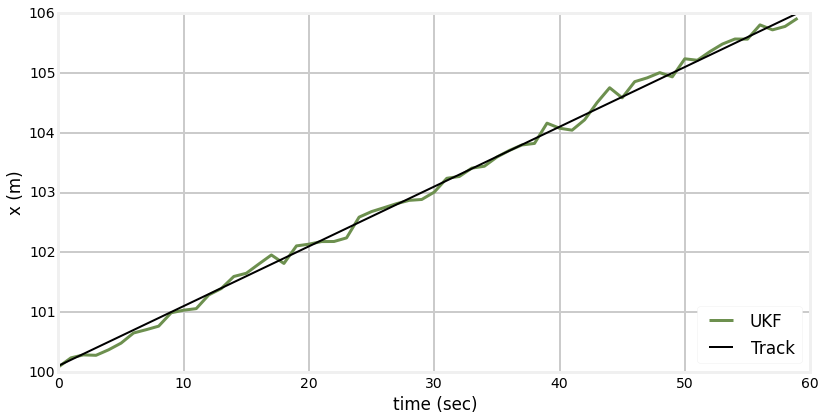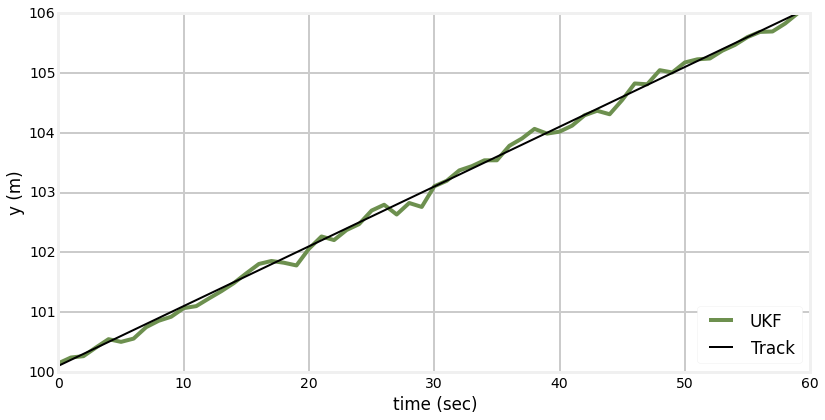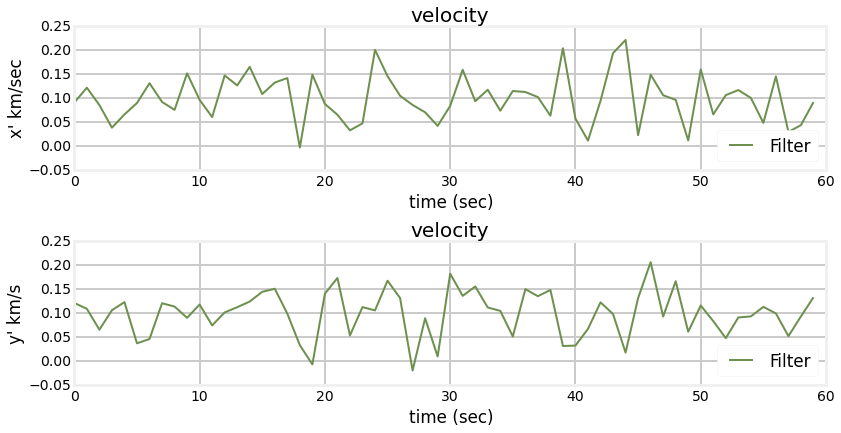In [ ]:


In [ ]:



## Sigma Point Algorithm¶

As I already said there are several published algorithms for choosing the sigma points. I will present the method first chosen by the inventors of the UKF.

Our first sigma point is always going to be the mean of our input. We will call this $\mathcal{X}_0$. So,

$$\mathcal{X}_0 = \mu$$

Tne corresponding weight for this sigma point is $$W_0 = \frac{\kappa}{n+\kappa}$$ where $n$ is the dimension of the problem, and $\kappa$ is a scaling factor that will be discussed in a moment.

The rest of the sigma points are defined to be

\begin{aligned} \mathcal{X}_i &= \mu + (\sqrt{(n+\kappa)\Sigma})_i\,\,\,\, &\text{for}\text{ i=1 .. n} \\ \mathcal{X}_i &= \mu - (\sqrt{(n+\kappa)\Sigma})_{i-n}\,\,\,\,\, &\text{for}\text{ i=(n+1) .. 2n} \\ \text{and the corresponding weights are} \\ W_i &= \frac{1}{2(n+\kappa)}\,\,\,\text{for i=1,2..2n} \end{aligned}

$\kappa$ (kappa) is a scaling factor that controls how far away from the mean we want the points to be. A larger kappa will choose points further away from the mean, and a smaller kappa will choose points nearer the mean. Julier and Uhlmann suggest using $\kappa + n = 3$ if the distribution is Gaussian, and perhaps choosing a different value if it is not Gaussian.

It may not be obvious why this is 'correct', and indeed, it cannot be proven that this is ideal for all nonlinear problems. But you can see that we are choosing the sigma points proportional to the square root of the covariance matrix, and the square root of variance is standard deviation. So, the sigma points are spread roughly according to 1 standard deviation. However, there is an $n$ term in there - the more dimensions there are the more the points will be spread out and weighed less.

## The Unscented Transform¶

The unscented transform is the core of the algorithm yet it is remarkably simple. Given a set of sigma points and a transfer function we need to compute the new mean and covariance of the points after they have passed through the function. In other words, loop through the sigma points and compute their new position after passing through your nonlinear function. Then just compute the mean and covariance of these points.

In other words, we just compute the mean and covariance from the equations above:

\begin{aligned} \mu &= \sum_i w_i\mathcal{X}_i\;\;\;&(2) \\ \Sigma &= \sum_i w_i{(\mathcal{X}_i-\mu)(\mathcal{X}_i-\mu)^\mathsf{T}}\;\;\;&(3) \end{aligned}

## The Unscented Filter¶

Now we can present the entire Unscented Kalman filter algorithm. Assume that there is a function $f(x)$ that performs the state transition for our filer - it predicts the next state given the current state. Also assume there is a measurement function $h(x)$ - it takes the current state and computes what measurement that state corresponds to. These are just nonlinear forms of the $\mathbf{F}$ and $\mathbf{H}$ matrices used by the linear Kalman filter.

### Predict Step¶

For the predict step, we will generate the weights and sigma points as specified above. \begin{aligned} \mathcal{X} &= sigma\_function(\mu, \Sigma) \\ W &= weight\_function(n, \kappa)\end{aligned}

We pass each sigma point through the function f.

$$\mathcal{X_f} = f(\mathcal{X})$$

Then we compute the predicted mean and covariance using the unscented transform. I've dropped the subscript $i$ for readability, and switched to the roman letters x and P for the mean and covariance.

\begin{aligned} \mathbf{x}^- &= \sum W\mathcal{X_f} \\ \mathbf{P}^- &= \sum W{(\mathcal{X_f}-x^-)(\mathcal{X_f}-x^-)^\mathsf{T}} + \mathbf{Q} \end{aligned}

This corresponds with the linear Kalman filter equations of \begin{aligned} \mathbf{x}^- &= \mathbf{Fx}\\ \mathbf{P}^- &= \mathbf{FPF}^T+\mathbf{Q} \end{aligned}

### Update Step¶

Now we can perform the update step of the filter. Recall that Kalman filters perform the update state in measurement space. So, the first thing we do is convert the sigma points into measurements using the $h(x)$ function.

$$\mathcal{X_z} = h(\mathcal{X})$$

Now we can compute the mean and covariance of these points using the unscented transform.

\begin{aligned} \mathbf{x}_z &= \sum w\mathcal{X_z} \\ \mathbf{P}_z &= \sum w{(\mathcal{X_z}-x)(\mathcal{X_z}-x)^\mathsf{T}} + \mathbf{R} \end{aligned}

The $z$ subscript denotes that these are the mean and covariance for the measurements.

All that is left is to compute the residual and Kalman gain.

The residual is trivial to compute:

$$\mathbf{y} = \mathbf{z} - \mathbf{x}_z$$

The Kalman gain is not much worse. We have to compute the cross variance of the state and the measurements, which we state without proof to be:

$$\mathbf{P}_{xz} =\sum W(\mathcal{X}-x)(\mathcal{X_z}-\mathbf{x}_z)^\mathsf{T}$$

And then the Kalman gain is defined as $$K = \mathbf{P}_{xz} \mathbf{P}_z^{-1}$$

Finally, we compute the new state estimate using the residual and Kalman gain:

$$\hat{\mathbf{x}} = \mathbf{x}^- + \mathbf{Ky}$$

and the new covariance is computed as:

$$\mathbf{P} = \mathbf{P}^- - \mathbf{PKP}_z\mathbf{K}^\mathsf{T}$$

This step contains a few equations you have to take on faith, but you should be able to see how they relate to the linear Kalman filter equations. We convert the mean and covariance into measurement space, add the measurement error into the measurement covariance, compute the residual and kalman gain, compute the new state estimate as the old estimate plus the residual times the Kalman gain, and then convert both back into state space. The linear algrebra is slightly different from the linear Kalman filter, but the algorithm is the same.

## Using the UKF¶

We are now ready to consider implementing a Kalman filter. All of the math is above is already implemented in FilterPy, and you are perhaps a bit lost at this point, so lets just launch into solving some problems so you can gain confidence in how each the UKF actually is. Later we will revisit how the UKF is implemented in Python.

Let's solve the 'bearing only' target tracking problem. Here the idea is that we have a ship or other platform tracking a moving target. Our sensor only provides the bearing to the target relative to the ship.

This is a nonlinear problem because the conversion between a target position and the bearing is:

$$\theta = \tan^{-1}\frac{y_{ship} - y_{target}}{x_{ship} - x_{target}}$$

If we were solving this problem using and EKF we would need to compute the Jacobian of this transform to linearize the filter. With the UKF we do not have to do this - we will see how this works as we work the example.

### Define State¶

First, lets define our filter state. Let's assume a constant velocity model for the target, so a reasonable state would be

$$\mathbf{x} = \begin{bmatrix}x\\ \dot{x} \\ y \\ \dot{y}\end{bmatrix}$$

where $x$ and $y$ are the position of the target, and $\dot{x}$ and $\dot{y}$ are its velocity.

### Define State Transition¶

Now we need to tell the filter how to perform the state transition - how to predict the next position from the current position. In the linear Kalman filter this is the equation $\mathbf{x}^- = \mathbf{Fx}$ but the unscented Kalman filter is intended for nonlinear problems. We cannot implement nonlinear behavior using linear algebra, so instead of providing the filter with a matrix we need to provide the filter with a function that computes the transition.

As it turns out our state transition is linear. We model the target movement using the standard Newtonian equation $$x_k = x_{k-1} + \dot{x}\Delta t$$

However, the UKF code in FilterPy does not differentiate between linear and nonlinear versions, so we will need to write a Python function to perform this computation. The signature of the function required by filterpy.UnscentedKalmanFilter is

def fx(x, dt):
return x



The filter implements the state as a one dimensional array, so our function can be implemented as

In :
def fx(x,dt):
# pos = pos + vel
# vel = vel
x += x
x += x
return x


FilterPy's KF and EKF code uses a 2D array to store the state. Due to implementation details the UKF uses a 1D array. I am not enamored of this inconistency, but for now this is how it is.

### Define the Measurement Function¶

Now we define our measurement function. In the linear KF this is implemented with the $\mathbf{H}$ matrix, but because the UKF is nonlinear we need to provide a function. We have already given the computation of the bearing from position as

$$\theta = \tan^{-1}\frac{y_{ship} - y_{target}}{x_{ship} - x_{target}}$$

so all we have to do is compute that in a function. The signature of this function is required to be

def hx(x):
return measurement



The filter state does not includes the ship's position, so we will assume a global variable ship_pos is defined that specifies the (x,y) coordinate for the ship.

In :
import math
def hx(x):
""" measurement function - convert position to bearing"""
return math.atan2(ship_pos - x, ship_pos - x)


And those two functions are the crux of the problem. All that remains is defining the noise in the system, and running the filter.

Important Note: There is a nonlinearity that we are not considering, the fact that angles are modular. Kalman filters operate by computing the differences between measurements. The difference between $359^\circ$ and $1^\circ$ is $2^\circ$, but a subtraction of the two values, as implemented by the filter, yields a value of $358^\circ$. This is exacerbated by the UKF which computes sums of weighted values in the unscented transform. For now we will place our sensors and targets in positions that avoid these nonlinear regions. Later in the chapter I will show you how to handle this problem.

Design Noise Parameters

We have spent a lot of time designing the $\mathbf{R}$, $\mathbf{Q}$, and $\mathbf{P}$ matrices in past chapters, and nothing changes for the UKF filter, so I will just choose some arbitrary values without much discussion. Let's assume the standard deviation in the bearing measurement is $\sigma=1^\circ$.

## Implement the Filter¶

So let's implement a filter given the design above. I'll just present the code; it should look very similar to the linear KF to you. The main difference is how we construct the filter:

f = UKF(dim_x=4, dim_z=1, dt=dt, fx=fx, hx=hx, kappa=0.)



When you construct the filter you have to provide the state transition function, the measurement function, and the value for $\kappa$ along with the usual dimension and time step values.

In :
from filterpy.kalman import UnscentedKalmanFilter as UKF
from filterpy.common import Q_discrete_white_noise

def fx(x,dt):
# pos = pos + vel
# vel = vel
x += x
x += x
return x

def bearing(ship_pos, target_pos):
return math.atan2(target_pos- ship_pos, target_pos - ship_pos)

def hx(x):
""" measurement function - convert position to bearing"""
return math.atan2(x - ship_pos, x - ship_pos)

dt = 0.1
ship_pos = [-40,20]
target_pos = [0,0]

f = UKF(4, 1, dt, fx=fx, hx=hx, kappa=0.)
f.x = np.array([target_pos, 0., target_pos, 0.])
Q = Q_discrete_white_noise(2, dt, .01)
f.Q[0:2,0:2] = Q
f.Q[2:4, 2:4] = Q

f.R *= std_noise**2
f.P *= 100

N = 300
xs = []
for i in range(N):
target_pos += 1 + randn()*0.0001 # add some process noise
angle = bearing(ship_pos, target_pos) + randn()*std_noise

f.predict()
f.update(angle)
xs.append(f.x)

xs = np.asarray(xs)
plt.plot([i/10 for i in range(N)], xs[:,0])
plt.xlabel('time')
plt.ylabel('target X position')
plt.show()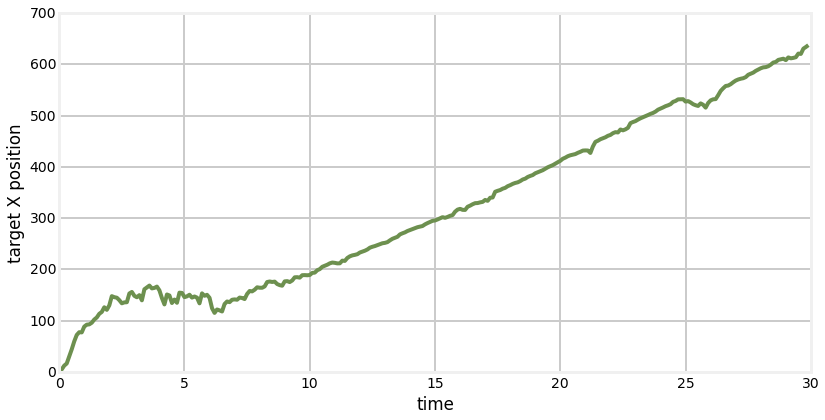If we look at only the target's estimated position in x, the results look very good. After running for several seconds the filter appears to settle down and produce a reasonably linear output for the target's position. However, let's look at the 2D plot of position.

In :
book_format.equal_axis()

plt.xlabel('target X position')
plt.ylabel('target Y position')
plt.plot(xs[:,0], xs[:,2])
plt.show()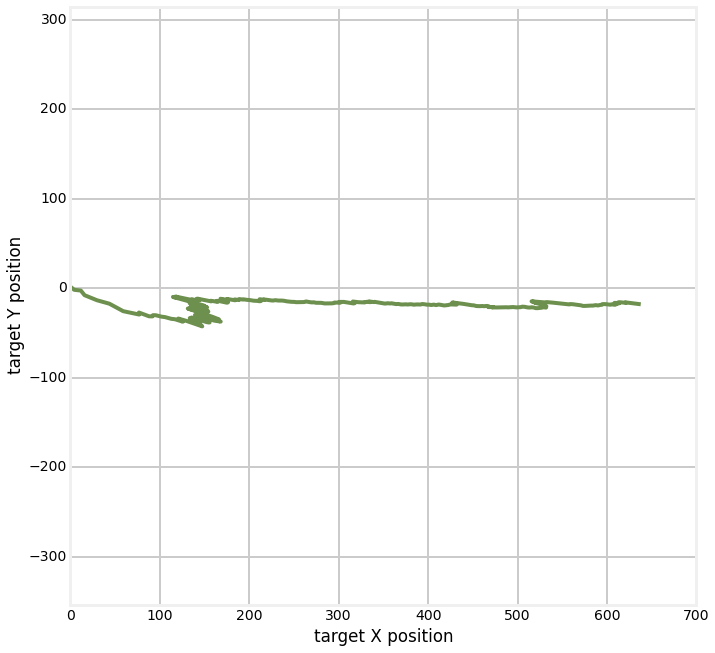As we can see the actual performance is abysmal. This is not an indictment of the UKF, but of the difficulty of the problem we are trying to solve. There are an infinite number of solutions for each measurement. For example, if our ship is at (0, 20), a bearing reading of 0 could reflect a target position of (0, 20) or (100, 20), or (1e300, 20). The Kalman filtering literature has many treatments of this problem; a common solution is to have the ship perform manuevers while tracking. This allows the filter to reduce the range of possibilities to something managable. This is rather outside the scope of this book so I will leave it to you to search the literature if you are interested in this specific problem.

Instead, I will make the problem tractable by introducing a second sensor. The problem is as follows. Assume we have two sensors, each which provides a bearing only measurement to the target, as in the chart below. In the chart the width of the circle is intended to denote a different amount of sensor noise.

In :
circle1=plt.Circle((-4,0),5,color='#004080',fill=False,linewidth=20, alpha=.7)
circle2=plt.Circle((4,0),5,color='#E24A33', fill=False, linewidth=5, alpha=.7)

fig = plt.gcf()
ax = fig.gca()

plt.axis('equal')
plt.xlim((-10,10))
plt.ylim((-10,10))

plt.plot ([-4,0], [0,3], c='#004080')
plt.plot ([4,0], [0,3], c='#E24A33')
plt.text(-4, -.5, "A", fontsize=16, horizontalalignment='center')
plt.text(4, -.5, "B", fontsize=16, horizontalalignment='center')

plt.show()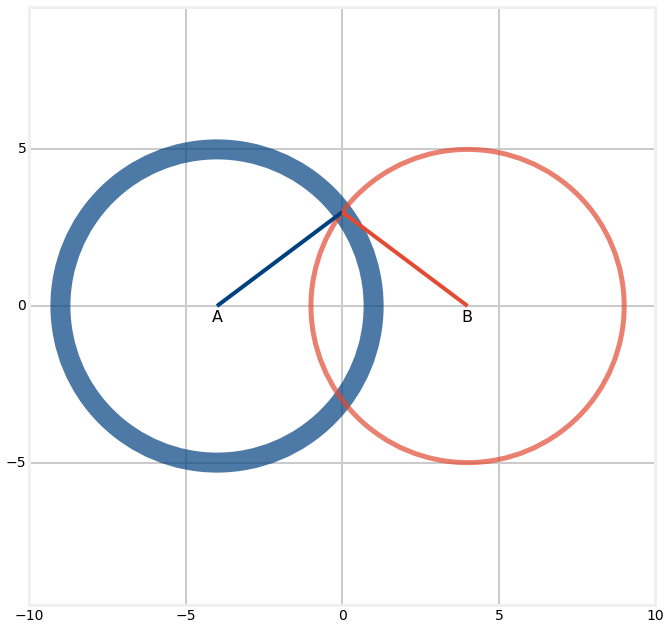We can compute the bearing between a sensor and the target as follows:

def bearing(sensor, target):
return math.atan2(target - sensor, target - sensor)

So our filter will need to receive a vector of 2 measurements during each update, one for each sensor. We can implement that as:

def measurement(A_pos, B_pos, pos):
angle_a = bearing(A_pos, pos)
angle_b = bearing(B_pos, pos)
return [angle_a, angle_b]

The design of the measurement function and state transition function can remain the same as nothing has changed that would affect them.

In :
from filterpy.kalman import UnscentedKalmanFilter as UKF
from filterpy.common import Q_discrete_white_noise

def bearing(sensor, target_pos):
return math.atan2(target_pos - sensor, target_pos - sensor)

def measurement(A_pos, B_pos, pos):
angle_a = bearing(A_pos, pos)
angle_b = bearing(B_pos, pos)
return [angle_a, angle_b]

def fx(x,dt):
x += x
x += x
return x

def hx(x):
# measurement to A
pos = (x, x)
return measurement(sa_pos, sb_pos, pos)

def moving_target_filter(std_noise):
dt = 0.1
f = UKF(dim_x=4, dim_z=2, dt=dt, hx=hx, fx=fx, kappa=2.)
f.x = np.array([target_pos, 1., target_pos, 1.])

Q = Q_discrete_white_noise(2, dt, 1.1)
f.Q[0:2,0:2] = Q
f.Q[2:4, 2:4] = Q

f.R *= std_noise**2
f.P *= 1000

return f

def plot_straight_line_target(f, std_noise):
xs = []
txs = []

for i in range(300):
target_pos += 1 + randn()*0.0001
target_pos += 1 + randn()*0.0001
txs.append((target_pos, target_pos))

z = measurement(sa_pos, sb_pos, target_pos)
z += randn() * std_noise
z += randn() * std_noise

f.predict()
f.update(z)
xs.append(f.x)

xs = np.asarray(xs)
txs = np.asarray(txs)

plt.plot(xs[:,0], xs[:,2])
plt.plot(txs[:,0], txs[:,1])
plt.show()

np.random.seed(123)

sa_pos = [-40, 0]
sb_pos = [40, 0]
target_pos = [40, 20]

f = moving_target_filter(std_noise)
plot_straight_line_target(f, std_noise)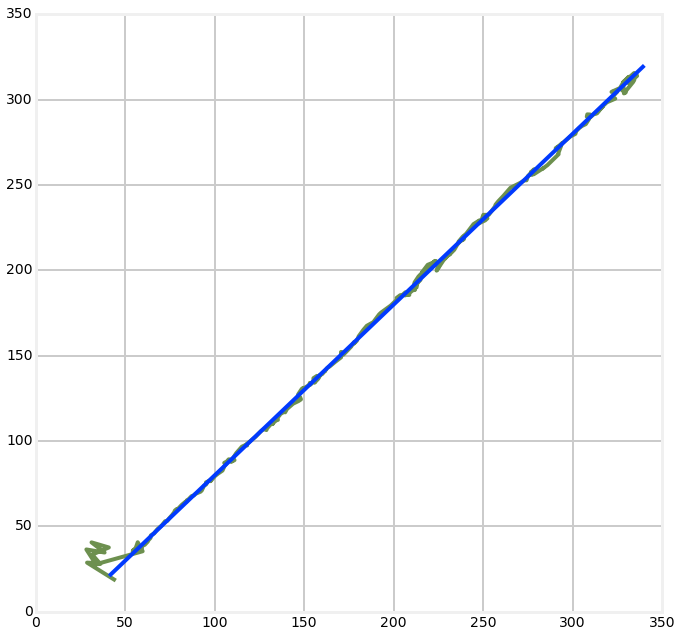This looks quite good to me. There is a very large error at the beginning of the track, but the filter is able to settle down and start producing good data.

Let's revisit the nonlinearity of the angles. I will position the target between the two sensors with the same y-coordinate. This will cause a nonlinearity in the computation of the sigma means and the residuals.

In :
np.random.seed(12330)
target_pos = [0, 0]
f = moving_target_filter(std_noise)
plot_straight_line_target(f, std_noise)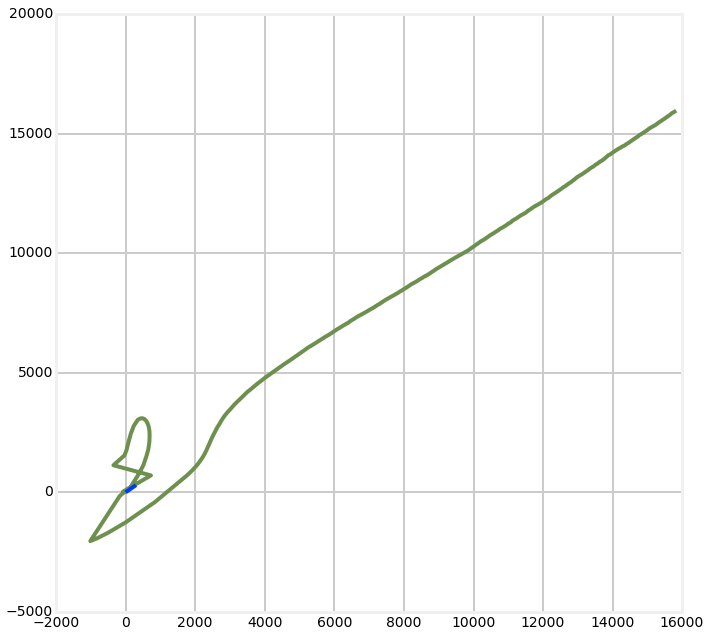If we seed the random number generator just right we can get the filter to eventually lock onto the signal and converge to a solution, but of course this is untenable behavior for a real world filter. I will deal with this nonlinearity later; I bring it up now in case you are experimenting with the code above and you start to see this sort of behavior.

## Exercise: Track a target moving in a circle¶

Change the simulated target movement to move in a circle. Avoid angular nonlinearities by putting the sensors well outside the movement range of the target, and avoid the angles $0^\circ$ and $180^\circ$.

In :
# your solution here


### Solution¶

We have a few choices here. First, if we know the movement of the target we can design our filter's state transition function so that it correctly predicts the circular movement. For example, suppose we were tracking a boat race optically - we would want to take track shape into account with our filter. However, in this chapter we have not been talking about such constrained behavior, so I will not build knowledge of the movement into the filter. So my implementation looks like this.

In :
def plot_circular_target(f, std_noise, target_pos):
xs = []
txs = []
for i in range(300):
txs.append((target_pos, target_pos))

z = measurement(sa_pos, sb_pos, target_pos)
z += randn() * std_noise
z += randn() * std_noise

f.predict()
f.update(z)
xs.append(f.x)

xs = np.asarray(xs)
txs = np.asarray(txs)

plt.plot(xs[:,0], xs[:,2])
plt.plot(txs[:,0], txs[:,1], linestyle='-.')
plt.axis('equal')
plt.show()

sa_pos = [-240, 200]
sb_pos = [240, 200]

np.random.seed(12283)
target_pos = [0, 0]
f = moving_target_filter(std_noise)
plot_circular_target(f, std_noise, target_pos)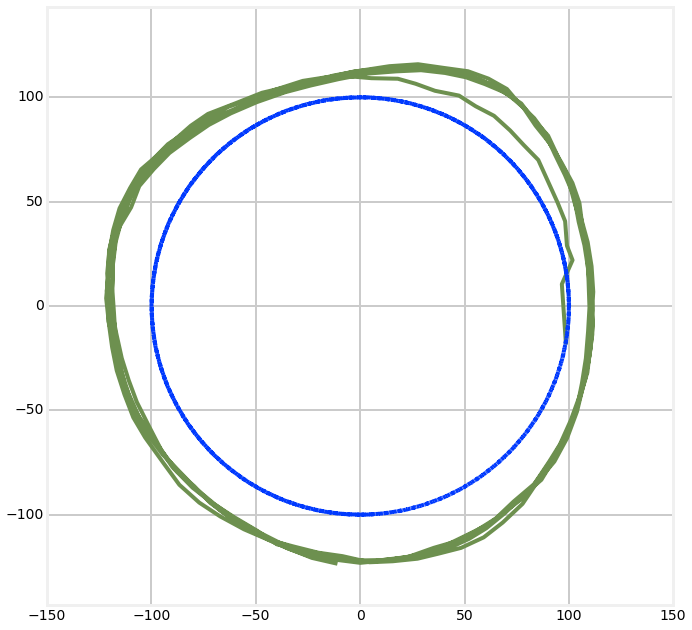### Discussion¶

The filter tracks the movement of the target, but never really converges on the track. This is because the filter is modeling a constant velocity target, but the target is anything but constant velocity. As mentioned above we could model the circular behavior by defining the fx() function, but then we would have problems when the target is not moving in a circle. Instead, let's tell the filter we are fare less sure about our process model by making $\mathbf{Q}$ much larger.

In :
def circular_target_filter(std_noise):
f = UKF(dim_x=4, dim_z=2, dt=0.1, hx=hx, fx=fx, kappa=2.)
f.x = np.array([target_pos, 1., target_pos, 1.])

Q = Q_discrete_white_noise(2, dt, 10.1)
f.Q[0:2,0:2] = Q
f.Q[2:4, 2:4] = Q

f.R *= std_noise**2
f.P *= 1000

return f

cf = circular_target_filter(std_noise)
target_pos = [0, 0]
plot_circular_target(cf, std_noise, target_pos)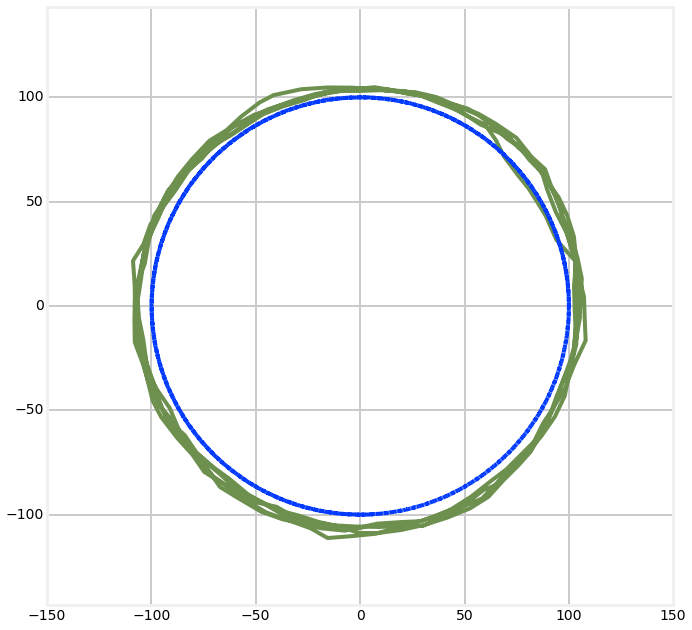The convergence is not perfect, but it is far better.

## Exercise: Sensor Position Effects¶

Is the behavior of the filter invariant for any sensor position? Find a sensor position that produces bad filter behavior.

In :
# your answer here


### Solution¶

We have already discussed the problem of angles being modal, so causing that problem by putting the sensors at y=0 would be a trivial solution. However, let's be more subtle than that. We want to create a situation where there are an infinite number of solutions for the sensor readings. We can achieve that whenever the target lies on the straight line between the two sensors. In that case there is no triangulation possible and there is no unique solution. My solution is as follows.

In :
std_noise = math.radians(0.5)
sa_pos = [-200,200]
sb_pos = [200,-200]
plt.scatter(*sa_pos, s=200)
plt.scatter(*sb_pos, s=200)
cf = circular_target_filter(std_noise)
target_pos = [0, 0]
plot_circular_target(cf, std_noise, target_pos)